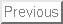Next: 1. Introduction

# AMIGOS: Analytical Model Interface & General Object-Oriented Solver

M. Radi, E. Leitner, and S. Selberherr
Institute for Microelectronics, TU Vienna
Gusshausstrasse 27-29, A-1040 Vienna, Austria
http://www.iue.tuwien.ac.at

### Abstract:

To accurately simulate modern semiconductor process steps, a simulation tool must include a variety of physical models and numerical methods. Increasingly complex physical formulations are required to account for effects that were not important in simulating previous generations of technology. Thus flexibility in definition of models as well as numerical solving methods is highly desirable. An object-oriented approach has been applied to implementing a dimension independent solver which uses an analytical input interface in the manner of Math-Cad, Mathematica, Matlab but highly optimized for numerical calculations of partial differential equations for complex simulation domains. It is equipped with a numerical solver, that supports direct and iterative solution methods, as well as an adaptive hierarchical grid adaptation algorithm which can be controlled by the analytical input language. To show the abilities of AMIGOS several examples in different dimensions are demonstrated.

M. Radi, E.Leitner, and S. Selberherr